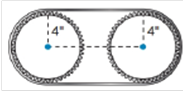Chapter 8.4, Problem 41EElementary Geometry For College St...

7th Edition
Alexander + 2 others
ISBN: 9781337614085

Solutions

Chapter
SectionElementary Geometry For College St...

7th Edition
Alexander + 2 others
ISBN: 9781337614085
Textbook Problem

In exercises 34-45, use your calculator value of π unless otherwise stated. Round answers to two decimal places.If two gears, each of radius 4 in are used in a chain drive system with a chain length of 54 in. What is the distance between the centers of the gears.To determine

To Find:

The distance between the centers of two gears

Explanation

The total chain length is 54 in.

The chain semi circularly covers both the gears.

Therefore, the chain forms a circle over the the gears together.

The radius of the gears is 4 in.

The circumference of the circular chain is C=2πr.

Here, π=3.1416.

Therefore, 23.14164=25.13

Still sussing out bartleby?

Check out a sample textbook solution.

See a sample solution

The Solution to Your Study Problems

Bartleby provides explanations to thousands of textbook problems written by our experts, many with advanced degrees!

Get Started

Simplify: 810

Elementary Technical Mathematics

Use Definition 8 to prove that limx1x=0. Definition 8

Single Variable Calculus: Early Transcendentals, Volume I

Explain in your own words the meaning of limxf(x)=L and limxf(x)=M.

Applied Calculus for the Managerial, Life, and Social Sciences: A Brief Approach

The directrix of the conic given by is:

Study Guide for Stewart's Multivariable Calculus, 8th

What rationalizing substitution should be made for 1x+x5dx? a) u = x b) u=x c) u=x5 d) u=x10

Study Guide for Stewart's Single Variable Calculus: Early Transcendentals, 8th